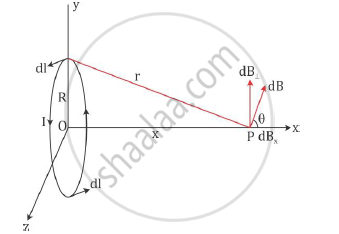# Deduce the Expression for the Magnetic Field at a Point on the Axis of a Current Carrying Circular Loop of Radius ‘R’ Distant ‘X’ from the Centre - Physics

Deduce the expression for the magnetic field at a point on the axis of a current carrying circular loop of radius ‘R’ distant ‘x’ from the centre. Hence, write the magnetic field at the centre of a loop.

#### Solution

dB∝ (IDlxxr)/r^3

dB =mu_0/(4pi) (Idlxxr)/r^3

Here, mu_0/(4pi)is a constant of proportionality.

The magnitude of this field is given as

|dB|=mu_0/(4pi) (Idlsintheta)/r^2

Magnetic field on the axis of a circular current loop:

Consider a circular loop carrying a steady current I. The loop is placed in the y–z plane
with its centre at origin O and has a radius R.Let x be the distance of point P from the centre of the loop where the magnetic field is to
be calculated. Consider a conducting element dl of the loop. The magnitude dB of the
magnetic field due to dl is given by the Biot–Savart’s law as

dB=mu_0/(4pi) (I|dlxxr|)/r^3

From the figure, we see that r2 = x2 + R2.

Any element of the loop will be perpendicular to the displacement vector from the element to the axial point. Hence, we have |dlxxr|=rdl Thus, we have

dB=mu_0/(4pi) (Idl)/r^2=mu_0/(4pi) (Idl)/(x^2+R^2) ".....(1)"

The direction of dB is perpendicular to the plane formed by dl and r. It has an xcomponent
dBx and a component perpendicular to x-axis dB⊥

The perpendicular components cancel each other when summed over. Therefore, only the x component contributes. The net contribution is obtained by integrating dBx = dB cosθ

From the figure, we see that

costheta=R/r=R/sqrt(x^2+R^2) "...(2)"

From equations (1) and (2), we get

dB_x=dBcostheta=mu_0/(4pi) (Idl)/(x^2+R^2)xxR/sqrt(x^2+R^2)=(mu_0Idl)/(4pi)xxR/(x^2+R^2)^(3/2)

The summation of dl yields circumference of the loop 2πR. Hence, the magnetic field at
point P caused by the entire loop is

B=B_xhati=(mu_0I(2piR))/(4pi)xxR/(x^2+R^2)^(3/2)hati

B=(mu_0IR^2)/(2(x^2+R^2)^(3/2))hati

Case: At the centre of the loop

At the centre x = o, so we have

B=(mu_0IR^2)/(2(R^2)^(3/2))hati=(mu_0IR^2)/(2R^3)hati=(mu_0I)/(2R)hati

Concept: Magnetic Force
Is there an error in this question or solution?